# MCAT Physical : Gibbs Free Energy

## Example Questions

← Previous 1

### Example Question #1 : Gibbs Free Energy

A scientist prepares an experiment to demonstrate the second law of thermodynamics for a chemistry class. In order to conduct the experiment, the scientist brings the class outside in January and gathers a cup of water and a portable stove.

The temperature outside is –10 degrees Celsius. The scientist asks the students to consider the following when answering his questions:

Gibbs Free Energy Formula:

ΔG = ΔH – TΔS

Liquid-Solid Water Phase Change Reaction:

H2O(l) ⇌ H2O(s) + X

The scientist prepares two scenarios.

Scenario 1:

The scientist buries the cup of water outside in the snow, returns to the classroom with his class for one hour, and the class then checks on the cup. They find that the water has frozen in the cup.

Scenario 2:

The scientist then places the frozen cup of water on the stove and starts the gas. The class finds that the water melts quickly.

After the water melts, the scientist asks the students to consider two hypothetical scenarios as a thought experiment.

Scenario 3:

Once the liquid water at the end of scenario 2 melts completely, the scientist turns off the gas and monitors what happens to the water. Despite being in the cold air, the water never freezes.

Scenario 4:

The scientist takes the frozen water from the end of scenario 1, puts it on the active stove, and the water remains frozen.

In scenario 1, the reaction of water freezing has which of the following quantities for the Gibbs Free Energy Formula?

Enthalpy: Negative; Entropy: Positive

Enthalpy: Negative; Entropy: Negative

Enthalpy: Positive; Entropy: Negative

Enthalpy: Positive; Entropy:  Positive

Enthalpy: Negative; Entropy: Zero

Enthalpy: Negative; Entropy: Negative

Explanation:

As water freezes, heat is released. The H value in the equation must therefore be negative.  Also as water freezes, there is a local decline in the amount of disorder. The S, or entropy, term in the Gibbs equation refers to entropy of the system. Thus, this term must be negative as well.

### Example Question #8 : Thermochemistry And Energetics

Diffusion can be defined as the net transfer of molecules down a gradient of differing concentrations. This is a passive and spontaneous process and relies on the random movement of molecules and Brownian motion. Diffusion is an important biological process, especially in the respiratory system where oxygen diffuses from alveoli, the basic unit of lung mechanics, to red blood cells in the capillaries.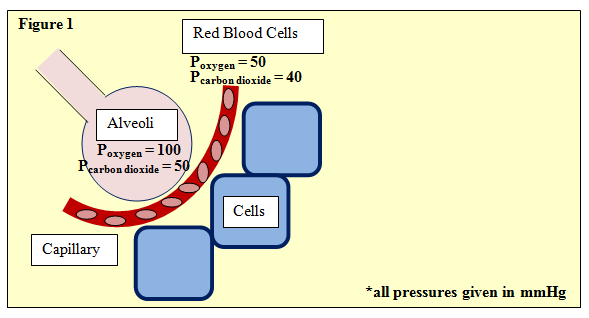Figure 1 depicts this process, showing an alveoli separated from neighboring cells by a capillary with red blood cells. The partial pressures of oxygen and carbon dioxide are given. One such equation used in determining gas exchange is Fick's law, given by:

ΔV = (Area/Thickness) · Dgas · (P1 – P2)

Where ΔV is flow rate and area and thickness refer to the permeable membrane through which the gas passes, in this case, the wall of the avlveoli. P1 and P2 refer to the partial pressures upstream and downstream, respectively. Further, Dgas­, the diffusion constant of the gas, is defined as:

Dgas = Solubility / (Molecular Weight)^(1/2)

In any diffusion process, what is ΔG, Gibbs energy?

ΔG = 0

ΔG ≤ 0

ΔG ≥ 0

Cannot be determined

ΔG ≤ 0

Explanation:

The key here is that diffusion, as mentioned in the passage, is a passive or spontaneous process. That is, it occurs without any energy expenditure, and therefore for any diffusion process, ΔG must be less than 0. In contrast, other transport mechanisms, such as active transport, require ATP as an energy source, and therefore ΔG ≥ 0.

### Example Question #9 : Thermochemistry And Energetics

A scientist is studying a reaction, and places the reactants in a beaker at room temperature. The reaction progresses, and she analyzes the products via NMR. Based on the NMR readout, she determines the reaction proceeds as follows: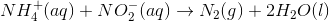In an attempt to better understand the reaction process, she varies the concentrations of the reactants and studies how the rate of the reaction changes. The table below shows the reaction concentrations as she makes modifications in three experimental trials.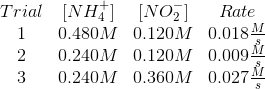After beginning the reaction described in the passage, a scientist waits as it comes to equilibrium at standard conditions. He calculates the equilibrium constant to be much greater than 1. Which of the following must be true?

The standard free energy change of the reaction is greater than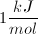The standard free energy change of the reaction is between zero andThe standard free energy change of the reaction is positive

The standard free energy change of the reaction is negative

The standard free energy change of the reaction is zero

The standard free energy change of the reaction is negative

Explanation:

If the equilibrium constant of a reaction is much greater than 1, its equilibrium is characterized by having a relative abundance of products to reactants.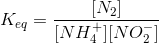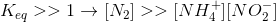This ratio implies that the reaction is spontaneous, and can be formally stated in terms of Gibbs free energy.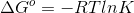### Example Question #10 : Thermochemistry And Energetics

Which of the following conditions will result in a spontaneous reaction?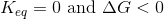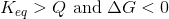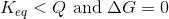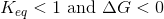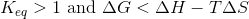Explanation:

This question tests two distinct, but related, principles: equilibrium constant and Gibbs free energy.

Equilibrium constant is given by the following equation, only when the concentrations represent equilibrium quantities of the compounds involved: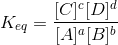In contrast, the reaction quotient is determined by this same equation when the compounds are not in equilibrium. What this means is that a reaction with a reaction quotient greater than the equilibrium constant at a given moment has an excess of products, compared to equilibrium. In contrast, a reaction with a reaction quotient less than the equilibrium constant has a relative abundance of reactions. In this later scenario, the reaction will proceed toward the products in order to achieve equilibrium.

An equilibrium constant greater than one indicates that there are more products than reactants at equilibrium, thus favoring the forward reaction and indicating spontaneity.

In summary, the equilibrium constant must be greater than the reaction quotient or greater than one in order for the reaction to be considered spontaneous.

Evaluating Gibbs free energy is much simpler: a negative value for Gibbs free energy indicates a spontaneous reaction. The equation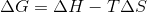is always true, and the inequality listed in one of the answer choices cannot exist.

### Example Question #11 : Thermochemistry And Energetics

A galvanic cell is set up, and is determined to have a standard cell potential of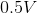. Which prediction must be true, based on this voltage?

Gibb's free energy is negative and the equilibrium constant is greater than one

The equilibrium constant is less than one

Electrons will flow toward the anode

The reaction is not spontaneous

Gibb's free energy is negative and the equilibrium constant is greater than one

Explanation:

There is an inherent relationship between cell potential, Gibb's free energy, and the equilibrium constant. Under standard conditions, a positive cell potential will signify a spontaneous reaction. This indicates that the Gibb's free energy (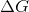) for the reaction must be negative. It also means that the equilibrium constant must be greater than one, indicating that the reaction favors the products over the reactants.

Electrons will always travel from the site of oxidation (anode) toward the site of reduction (cathode), regardless of reaction spontaneity.

### Example Question #1 : Gibbs Free Energy

A scientist prepares an experiment to demonstrate the second law of thermodynamics for a chemistry class. In order to conduct the experiment, the scientist brings the class outside in January and gathers a cup of water and a portable stove.

The temperature outside is –10 degrees Celsius. The scientist asks the students to consider the following when answering his questions:

Gibbs Free Energy Formula:

ΔG = ΔH – TΔS

Liquid-Solid Water Phase Change Reaction:

H2O(l) ⇌ H2O(s) + X

The scientist prepares two scenarios.

Scenario 1:

The scientist buries the cup of water outside in the snow, returns to the classroom with his class for one hour, and the class then checks on the cup. They find that the water has frozen in the cup.

Scenario 2:

The scientist then places the frozen cup of water on the stove and starts the gas. The class finds that the water melts quickly.

After the water melts, the scientist asks the students to consider two hypothetical scenarios as a thought experiment.

Scenario 3:

Once the liquid water at the end of scenario 2 melts completely, the scientist turns off the gas and monitors what happens to the water. Despite being in the cold air, the water never freezes.

Scenario 4:

The scientist takes the frozen water from the end of scenario 1, puts it on the active stove, and the water remains frozen.

Which of the following statements is true of scenario 3?

It is spontaneous at all temperatures.

It is never spontaneous.

It can be used to drive unfavorable reactions.

It is spontaneous only at high temperatures.

It is spontaneous only at low temperatures.

It is never spontaneous.

Explanation:

The reaction described in scenario 3 is the persistence of fully liquid water in the face of low temperatures. In other words, the Liquid-Solid Water Phase Change Reaction is far to the left.  As X must be heat, and there is a shortage of heat in the cold air, the reaction would have to be driven to the right via Le Chatlier's Principle. It could never be spontaneous to the left.

### Example Question #13 : Thermochemistry And Energetics

A scientist prepares an experiment to demonstrate the second law of thermodynamics for a chemistry class. In order to conduct the experiment, the scientist brings the class outside in January and gathers a cup of water and a portable stove.

The temperature outside is –10 degrees Celsius. The scientist asks the students to consider the following when answering his questions:

Gibbs Free Energy Formula:

ΔG = ΔH – TΔS

Liquid-Solid Water Phase Change Reaction:

H2O(l) ⇌ H2O(s) + X

The scientist prepares two scenarios.

Scenario 1:

The scientist buries the cup of water outside in the snow, returns to the classroom with his class for one hour, and the class then checks on the cup. They find that the water has frozen in the cup.

Scenario 2:

The scientist then places the frozen cup of water on the stove and starts the gas. The class finds that the water melts quickly.

After the water melts, the scientist asks the students to consider two hypothetical scenarios as a thought experiment.

Scenario 3:

Once the liquid water at the end of scenario 2 melts completely, the scientist turns off the gas and monitors what happens to the water. Despite being in the cold air, the water never freezes.

Scenario 4:

The scientist takes the frozen water from the end of scenario 1, puts it on the active stove, and the water remains frozen.

If a spontaneous reaction is harnessed to do work, how much useful work can the reaction do?

Spontaneous reactions typically proceed too quickly to do useful work

Exactly its Gibbs Free Energy value

Less than its Gibbs Free Energy value, as energy some must go to increase the entropy of the universe

Only nonspontaneous reactions coupled to secondary reactions can do useful work

Greater than its Gibbs Free Energy value, as this value does not consider enthalpy contributions

Exactly its Gibbs Free Energy value

Explanation:

The definition of Gibbs Free Energy is the amount of useful work that a reaction is able to do under defined conditions. It considers the amount of energy that is lost forever to the universe to comply with the Second Law of Thermodynamics.

### Example Question #14 : Thermochemistry And Energetics

ATP stores energy through its ability to lose a phosphate group to form ADP in the following reaction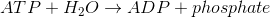At body temperature, the thermodynamic values for ATP hydrolysis are as follow.
ΔG = –30kJ/mol
ΔH = –20kJ/mol
ΔS = 34kJ/mol

Glycolysis is an energy-liberating process (ΔG = –218kJ/mol, ΔH = –20 kJ/mol) that is coupled with the conversion of two ADP molecules into two ATP molecules according to the following reaction.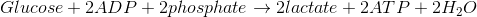What is the value of ΔG for the reaction below?–278kJ/mol

–218kJ/mol

218kJ/mol

–158kJ/mol

–158kJ/mol

Explanation:

First, recognize that if ΔG for the formation of ADP is –30 kJ/mol, the ΔG for the formation of ATP must be +30 kJ/mol. Next, we can combine the ΔG of glycolysis with the ΔG of the formation of two ATP molecules (notice the coefficient of 2 in order to balance ATP in the equation).

ΔGglucose = –218kJ/mol
ΔGATP = 30kJ/mol
ΔGtotal = –218 kJ/mol + 2(30kJ/mol) = –158kJ/mol

### Example Question #15 : Thermochemistry And Energetics

For any given chemical reaction, one can draw an energy diagram. Energy diagrams depict the energy levels of the different steps in a reaction, while also indicating the net change in energy and giving clues to relative reaction rate.

Below, a reaction diagram is shown for a reaction that a scientist is studying in a lab. A student began the reaction the evening before, but the scientist is unsure as to the type of the reaction. He cannot find the student’s notes, except for the reaction diagram below.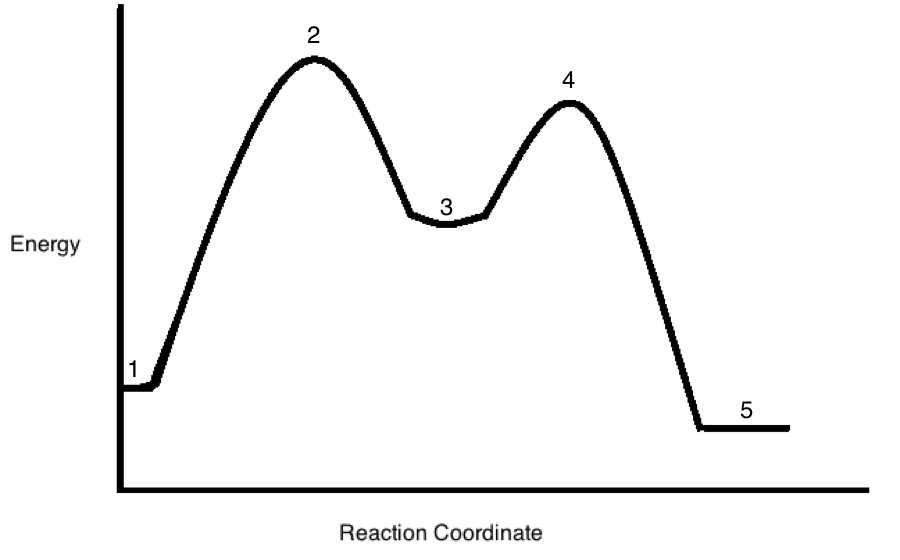Instead of "Energy" on the Y-axis, the graduate student in the passage instead labels the axis "Heat." In this case, what can we say for sure about the reaction in question?

(Note: consider the reaction in the vessel to be the system, and the remainder of the universe as the surroundings)

The system may either lose or gain entropy

The surroundings must lose entropy

The system must lose entropy

The system must gain entropy

There is no net entropy change in the system or the surroundings

The system may either lose or gain entropy

Explanation:

With the change to the chart described in the passage, we know that the system is losing heat from reactants to products (5 is lower than 1). This heat is going out of the system to the surroundings, increasing entropy of the universe. Because of this, the system may either gain or lose entropy.

Any entropy losses in the system, however, must be more than balanced by the entropy gained via heat in the surroundings. The universe (system + surroundings) always has a net gain of entropy. Essentailly, since the value for the surroundings will be positive, the value for the system may be either positive or negative, as long at their sum remains positive.

### Example Question #16 : Thermochemistry And Energetics

Imagine there is an endothermic reaction where entropy is increased in the system. This reaction is not spontaneous at room temperature. What can be done in order to make the reaction spontaneous?

Increase the concentration of the products

Increase the temperature

Decrease the reactant concentrations

Increase the temperature

Explanation:

The Gibbs free energy equation is written as.

Since a negativeresults in a spontaneous reaction, we can manipulate the variables in order to make the reaction progress. Since entropy is positive in this equation, an increasing temperature will eventually equal the positive enthalpy of the reaction. Once the temperature overtakes the enthalpy, the reaction will be spontaneous.

In order to be spontaneous, in this case,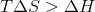.

← Previous 1

### All MCAT Physical Resources